How to compare two columns in Excel? - KING OF EXCELKINGEXCEL.INFO ( KING OF EXCEL ) Welcome KINGEXCEL.INFO - Nothing Is Unable ... About Excel Tricks, Learning VBA Programming, Dedicated Software, Accounting, Living Skills ...

## Wednesday, January 22, 2020

How to compare two columns in Excel?
The one query that I get a lot is – ‘how to compare two columns in Excel?’.
This can be done in many different ways, and the method to use will depend on the data structure and what the user wants from it.
For example, you may want to compare two columns and find or highlight all the matching data points (that are in both the columns), or only the differences (where a data point is in one column and not in the other), etc.
Since I get asked about this so much, I decided to write this massive tutorial with an intent to cover most (if not all) possible scenarios.
If you find this useful, do pass it on to other Excel users.
This Tutorial Covers:

Note that the techniques to compare columns shown in this tutorial are not the only ones.
Based on your dataset, you may need to change or adjust the method. However, the basic principles would remain the same.
If you think there is something that can be added to this tutorial, let me know in the comments section

## Compare Two Columns For Exact Row Match

This one is the simplest form of comparison. In this case, you need to do a row by row comparison and identify which rows have the same data and which ones does not.

### Example: Compare Cells in the Same Row

Below is a data set where I need to check whether the name in column A is the same in column B or not.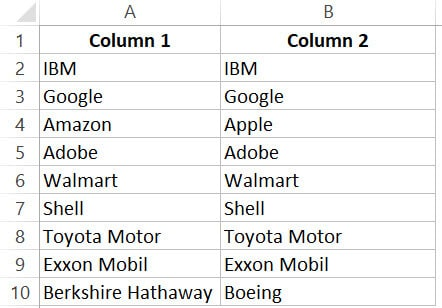If there is a match, I need the result as “TRUE”, and if doesn’t match, then I need the result as “FALSE”.
The below formula would do this:
`=A2=B2`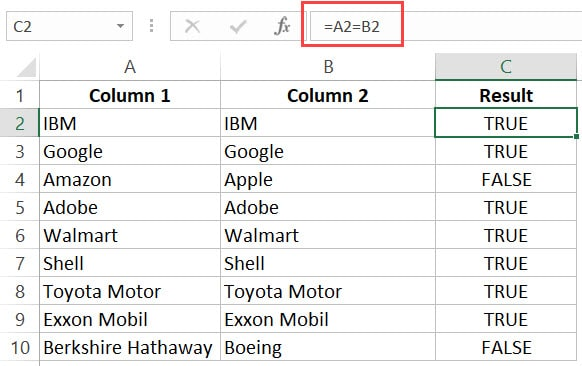### Example: Compare Cells in the Same Row (using IF formula)

If you want to get a more descriptive result, you can use a simple IF formula to return “Match” when the names are the same and “Mismatch” when the names are different.
`=IF(A2=B2,"Match","Mismatch")`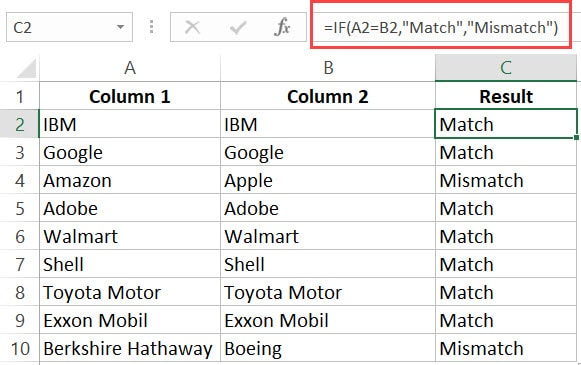Note: In case you want to make the comparison case sensitive, use the following IF formula:
`=IF(EXACT(A2,B2),"Match","Mismatch")`
With the above formula, ‘IBM’ and ‘ibm’ would be considered two different names and the above formula would return ‘Mismatch’.

### Example: Highlight Rows with Matching Data

If you want to highlight the rows that have matching data (instead of getting the result in a separate column), you can do that by using Conditional Formatting.
Here are the steps to do this:
1. Select the entire dataset.
2. Click the ‘Home’ tab.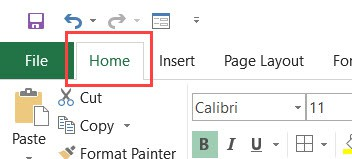3. In the Styles group, click on the ‘Conditional Formatting’ option.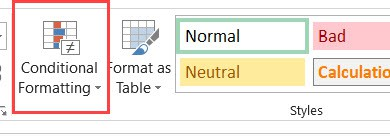4. From the drop-down, click on ‘New Rule’.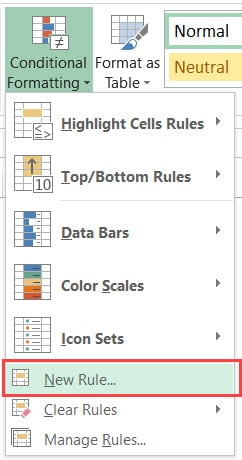5. In the ‘New Formatting Rule’ dialog box, click on the ‘Use a formula to determine which cells to format’.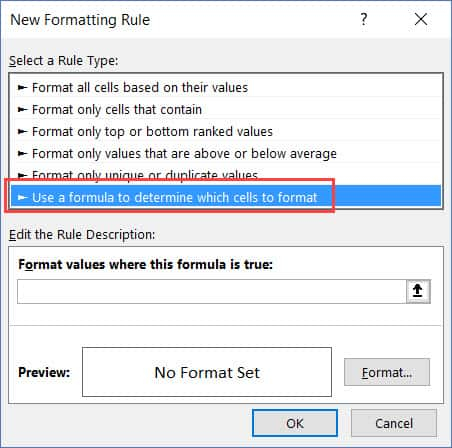6. In the formula field, enter the formula: =\$A1=\$B1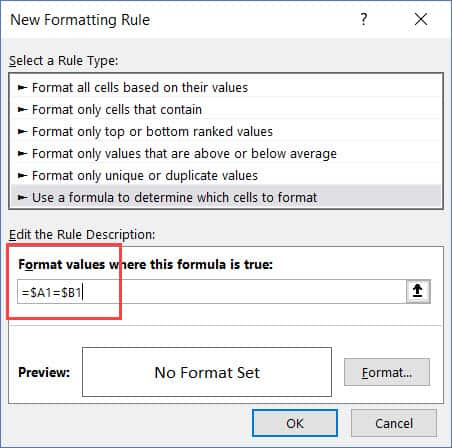7. Click the Format button and specify the format you want to apply to the matching cells.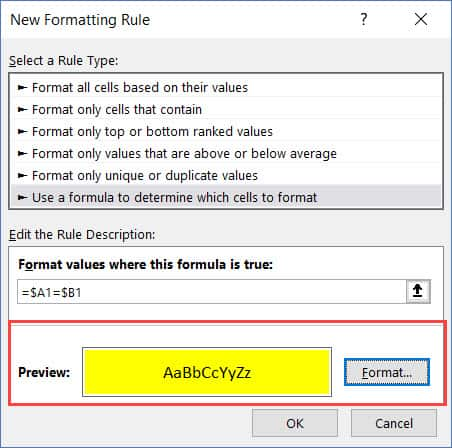8. Click OK.
This will highlight all the cells where the names are the same in each row.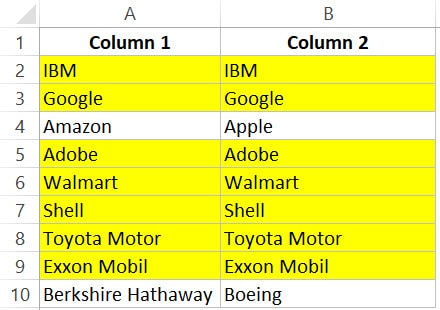## Compare Two Columns and Highlight Matches

If you want to compare two columns and highlight matching data, you can use the duplicate functionality in conditional formatting.
Note that this is different than what we have seen when comparing each row. In this case, we will not be doing a row by row comparison.

### Example: Compare Two Columns and Highlight Matching Data

Often, you’ll get datasets where there are matches, but these may not be in the same row.
Something as shown below: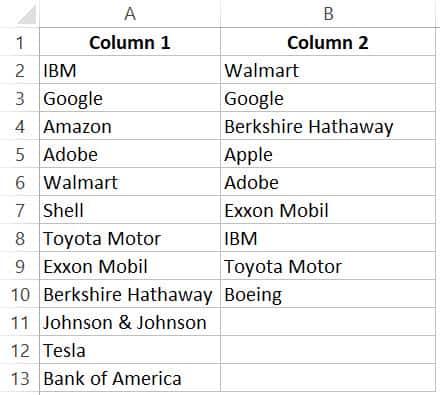Note that the list in column A is bigger than the one in B. Also some names are there in both the lists, but not in the same row (such as IBM, Adobe, Walmart).
If you want to highlight all the matching company names, you can do that using conditional formatting.
Here are the steps to do this:
1. Select the entire data set.
2. Click the Home tab.
3. In the Styles group, click on the ‘Conditional Formatting’ option.4. Hover the cursor on the Highlight Cell Rules option.
5. Click on Duplicate Values.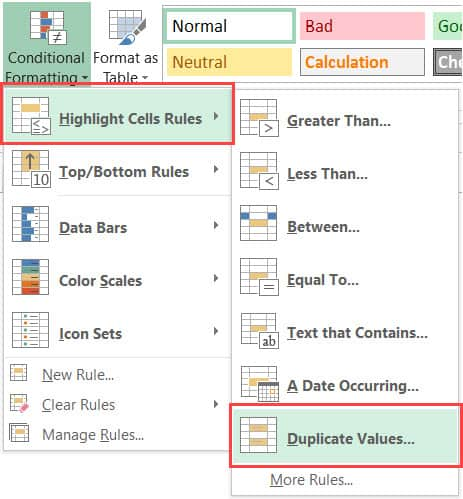6. In the Duplicate Values dialog box, make sure ‘Duplicate’ is selected.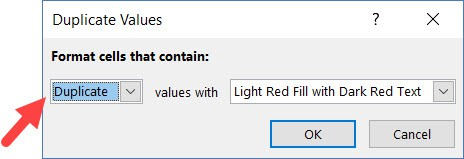7. Specify the formatting.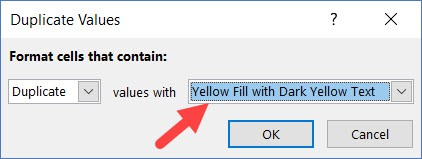8. Click OK.
The above steps would give you the result as shown below.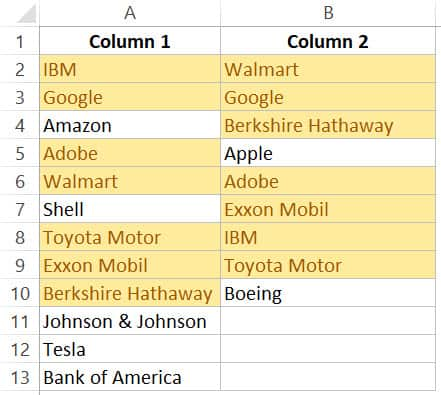Note: Conditional Formatting duplicate rule is not case sensitive. So ‘Apple’ and ‘apple’ are considered the same and would be highlighted as duplicates.

### Example: Compare Two Columns and Highlight Mismatched Data

In case you want to highlight the names which are present in one list and not the other, you can use the conditional formatting for this too.
1. Select the entire data set.
2. Click the Home tab.
3. In the Styles group, click on the ‘Conditional Formatting’ option.4. Hover the cursor on the Highlight Cell Rules option.
5. Click on Duplicate Values.6. In the Duplicate Values dialog box, make sure ‘Unique’ is selected.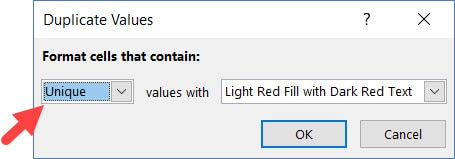7. Specify the formatting.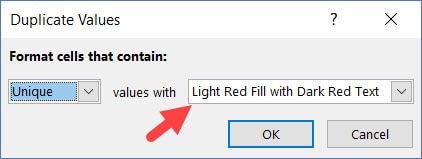8. Click OK.
This will give you the result as shown below. It highlights all the cells that have a name that is not present on the other list.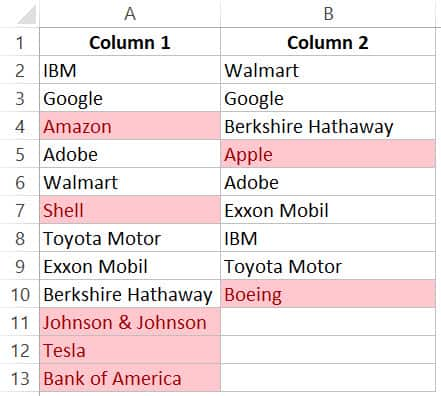## Compare Two Columns and Find Missing Data Points

If you want to identify whether a data point from one list is present in the other list, you need to use the lookup formulas.
Suppose you have a dataset as shown below and you want to identify companies that are present in column A but not in Column B,To do this, I can use the following VLOOKUP formula.
`=ISERROR(VLOOKUP(A2,\$B\$2:\$B\$10,1,0))`
This formula uses the VLOOKUP function to check whether a company name in A is present in column B or not. If it is present, it will return that name from column B, else it will return a #N/A error.
These names which return the #N/A error are the ones that are missing in Column B.
ISERROR function would return TRUE if there is the VLOOKUP result is an error and FALSE if it isn’t an error.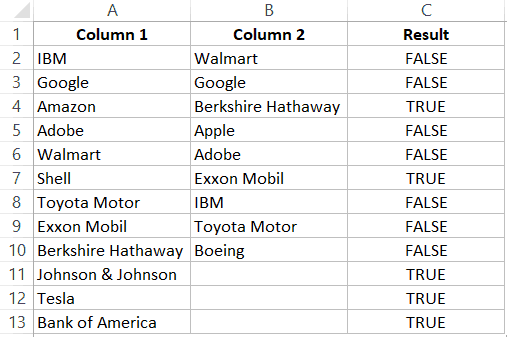If you want to get a list of all the names where there is no match, you can filter the result column to get all cells with TRUE.
You can also use the MATCH function to do the same;
`=NOT(ISNUMBER(MATCH(A2,\$B\$2:\$B\$10,0)))`
Note: Personally, I prefer using the Match function (or the combination of INDEX/MATCH) instead of VLOOKUP. I find it more flexible and powerful. You can read the difference between Vlookup and Index/Match here.

## Compare Two Columns and Pull the Matching Data

If you have two datasets and you want to compare items in one list to the other and fetch the matching data point, you need to use the lookup formulas.

### Example: Pull the Matching Data (Exact)

For example, in the below list, I want to fetch the market valuation value for column 2. To do this, I need to look up that value in column 1 and then fetch the corresponding market valuation value.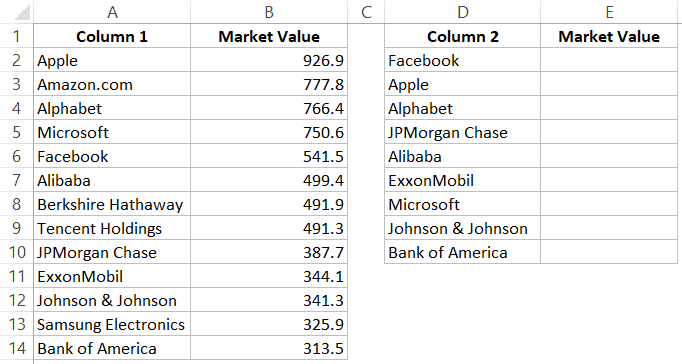Below is the formula that will do this:
`=VLOOKUP(D2,\$A\$2:\$B\$14,2,0)`
or
`=INDEX(\$A\$2:\$B\$14,MATCH(D2,\$A\$2:\$A\$14,0),2)`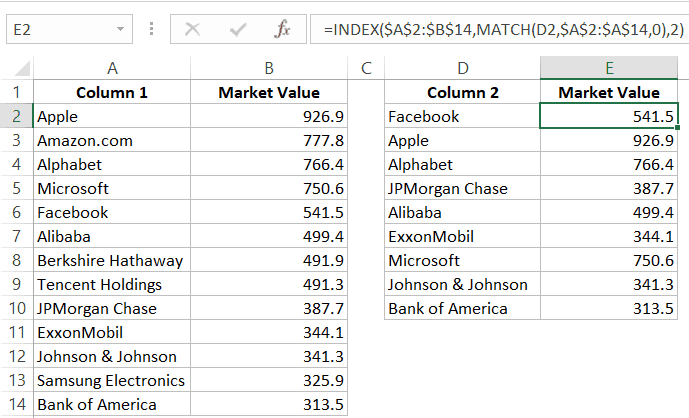### Example: Pull the Matching Data (Partial)

In case you get a dataset where there is a minor difference in the names in the two columns, using the above-shown lookup formulas is not going to work.
These lookup formulas need an exact match to give the right result. There is an approximate match option in VLOOKUP or MATCH function, but that can’t be used here.
Suppose you have the data set as shown below. Note that there are names that are not complete in Column 2 (such as JPMorgan instead of JPMorgan Chase and Exxon instead of ExxonMobil).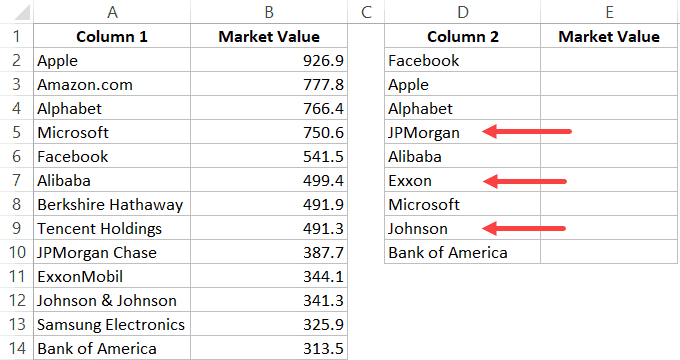In such a case, you can use a partial lookup by using wildcard characters.
The following formula will give is the right result in this case:
`=VLOOKUP("*"&D2&"*",\$A\$2:\$B\$14,2,0)`
or
`=INDEX(\$A\$2:\$B\$14,MATCH("*"&D2&"*",\$A\$2:\$A\$14,0),2)`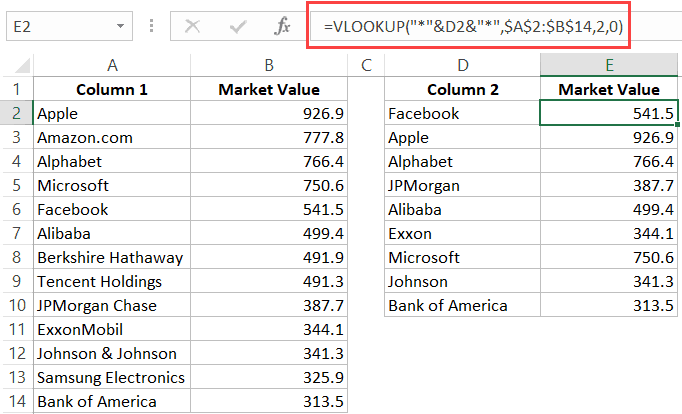In the above example, asterisk (*) is a wildcard character that can represent any number of characters. When the lookup value is flanked with it on both sides, any value in Column 1 which contains the lookup value in Column 2 would be considered as a match.
For example, *Exxon* would be a match for ExxonMobil (as * can represent any number of characters).
#evba #etipfree #kingexcel
📤You download App EVBA.info installed directly on the latest phone here : https://www.evba.info/p/app-evbainfo-setting-for-your-phone.html?m=1